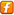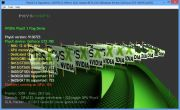Home Utilities Tutorials 3D Demos Graphics Cards Forums About

 GeeXLab
Current version: 0.45.1
>GeeXLab homepage

 FurMark
Current version: 1.30.0
>FurMark homepage

 GPU Caps Viewer
Current version: 1.55.0.0
>GPU Caps Viewer homepage

 GPU Shark
Current version: 0.26.0.0
>GPU Shark homepage

 Blogs
>JeGX's HackLab

 Geeks3D's Articles
>GPU Memory Speed Demystified

>How to Get your Multi-core CPU Busy at 100%

>How To Make a VGA Dummy Plug

>Night Vision Post Processing Filter

 PhysX FluidMark
Current version: 1.5.4
>FluidMark homepage

 TessMark
Current version: 0.3.0
>TessMark homepage

Current version: 0.3.0

 Demoniak3D
Current Version: 1.23.0
>Demoniak3D
>Libraries and Plugins
>Demos
>Codes SamplesLighting with GLSL
Phong Model

By J�r�me GUINOT aka 'JeGX' - jegx [at] ozone3d [dot] net

Initial draft: February 19, 2006
Update: March 8, 2006

[ Index ]

�Next Page||||1 - Summary of the Phong Lighting Equation2 - Point Light in GLSL3 - Spot Light in GLSL4 - Lighting Attenuation5 - Further Resources6 - Downloads

1 - Summary of the Phong Lighting EquationThe final color of the pixel displayed is given by the following equation:

`If = Ia + Id + Is`

where If is the intensity of the pixel final color, Ia is the intensity of the ambient color, Id is the intensity of the diffuse color and Is the intensity of the specular color. Ia, Id and Is are all four-dimensional RGBA vectors.

The Ia term is the ambient component. Ia is the result of the multiplication between the ambient component of the light and the ambient component of the material which composes the surface of the 3d object:

`Ia = (Al * Am) + (As * Am)`

where Al is the ambient component of the light and Am the ambient component of the material. Ia is generally a constant RGBA vector, and can even be pre-computed for the shader (this value is the same one independently from the pixel). A more advanced expression of this ambient term could be given by the Ambient Occlusion Lighting technique.

In OpenGL terms, As is defined as:

```float As = {0.1f, 0.1f, 0.1f, 1.0f };
glLightModelfv( GL_LIGHT_MODEL_AMBIENT, As );```

With Demoniak3D, As is defined in the scene node:

```<scene>
<global_ambient_light r="0.1" g="0.1" b="0.1" a="1.0" />
</scene>```

The Id term expresses the final diffuse component. This component is given by the following equation:

`Id = Dl * Dm * LambertTerm`

where Dl is the diffuse component of the light and Dm the diffuse component of the material. The LambertTerm factor is the keystone of the lighting equations. It is actually the value of this factor which will make it possible to create the self shadow of a 3d object (self-shadowing). This Lambert coefficient is calculated with the following dot product:

`LambertTerm = dot(N, L)`

where N is the normal vector to the considered pixel and L the light vector at the same pixel. This simple relation but so fundamental, tells us that the value of the Lambert coefficient will be maximum (i.e. equals to 1.0) if the angle between the two vectors (L and N) equals zero, i.e. if the pixel is directly in front of the light. For all the other cases, the Lambert coefficient will vary between 0.0 and 1.0 what will generate the self shadow.

The Is term expresses the final specular component. This component is obtained by:

`Is = Sm x Sl x pow( max(R dot E, 0.0), f )`

The Is term is from far the most complicated to calculate but it is responsible of these famous specular reflexions on the surface of the objects. Sl is the specular component of the light and Sm the specular component of the material. E the view vector or camera vector and R is the reflected light vector. R is obtained with:

`R = reflect(-L, N)`

where N is the normal vector to the pixel considered, and L the light vector (the same than Lambert coefficient) and reflect() a function (available in GLSL) which makes it possible to calculate the reflexion vector of L in relation to N. One implementation of reflect() could be:

`R = 2 * ( N dot L) * N - L`

The pow() function is the power function which makes it possible to raise a number n to the power of p: pow(n, p). f is the specular exponential factor (the famous shininess in OpenGL) which represents the hardness and the precision of the specular reflexion.

At the OpenGL level, the light's ambient, diffuse and specular terms are defined by:

```float Al = {0.0f, 0.0f, 0.0f, 1.0f };
glLightfv( GL_LIGHT0, GL_AMBIENT, Al );

float Dl = {1.0f, 1.0f, 1.0f, 1.0f };
glLightfv( GL_LIGHT0, GL_DIFFUSE, Dl );

float Sl = {1.0f, 1.0f, 1.0f, 1.0f };
glLightfv( GL_LIGHT0, GL_SPECULAR, Sl );
```

And in Demoniak3D terms:

```<light name="Light_01">
<ambient r="0.0" g="0.0" b="0.0" a="1.0" />
<diffuse r="1.0" g="1.0" b="1.0" a="1.0" />
<specular r="1.0" g="1.0" b="1.0" a="1.0" />
</light>```

At the OpenGL level, the material's ambient, diffuse and specular terms are defined by:

```float Am = {0.3f, 0.3f, 0.3f, 1.0f };
glMaterialfv(GL_FRONT_AND_BACK, GL_AMBIENT, Am );

float Dm = {0.9f, 0.5f, 0.5f, 1.0f };
glMaterialfv(GL_FRONT_AND_BACK, GL_DIFFUSE, Dm );

float Sm = {0.6f, 0.6f, 0.6f, 1.0f };
glMaterialfv(GL_FRONT_AND_BACK, GL_SPECULAR, Sm );

float f = 60.0f;
glMaterialf(GL_FRONT_AND_BACK, GL_SHININESS, f );
```

And for Demoniak3D:

```<material name="mat_torus" >
<ambient r="0.3" g="0.3" b="0.3" a="1.0" />
<diffuse r="0.9" g="0.5" b="0.5" a="1.0" />
<specular r="0.6" g="0.6" b="0.6" a="1.0" exp="60.0" />
</material>```

[ Index ]

�Next Page

 GeeXLab demosGLSL - Mesh exploderPhysX 3 cloth demoNormal visualizer with GS